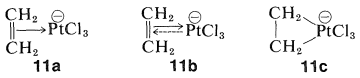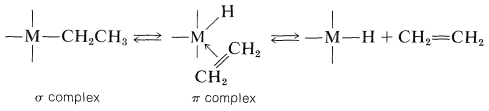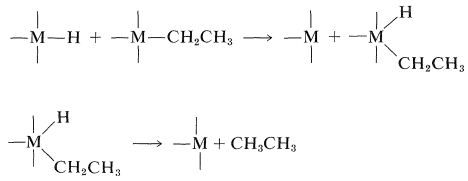# 31.2: Other Organometallic Compounds of Transition Metals

## $$\pi$$-Type Alkene and Cycloalkene Complexes

Not all organometallic compounds of transition metals date from the discovery of ferrocene. Many have been known for a long time but their structures were not understood. A conspicuous example is the anion of a substance known as Zeise's salt, which is formed from the reaction of ethanol with chloroplatinic acid, $$\ce{H_2PtCl_6}$$. The anion has the formula $$\ce{Pt(C_2H_4)Cl_3^-}$$. Although known since 1830, it finally was shown to have a structure with both ethene carbons bonded to platinum. The carbon-to-metal bonding usually is formulated as a $$\pi$$ complex, $$11a$$, or charge-transfer complex (Section 22-4D). Alternatively, we can think of the bonding in $$11a$$ as between platinum as a Lewis acid (electron-pair acceptor) and ethene as a Lewis base (electron-pair donor):The arrow in $$11a$$ symbolizes donation of $$\pi$$ electrons. However, because the stability of the ion is much greater than would be expected for either a simple acid-base or charge-transfer complex, it is postulated that unshared $$d$$ electrons from the metal participate in the bonding. This is symbolized by the dashed arrow in $$11b$$, which stands for donation of $$d$$ electrons into the $$\pi^*$$ orbital of the double bond or, as it is often called, "back bonding". Perhaps most simple is $$11c$$, where the $$\ce{C-Pt}$$ bonding is formulated as a three-membered ring with essentially $$\ce{C-Pt}$$ (\sigma\) bonds. In this formulation, full participation of a platinum electron pair is assumed.

Many complexes of alkenes, cycloalkenes, alkynes, and cycloalkynes with transition metals are now known. Some examples are:Many substances of this type have potential synthetic usefulness as catalysts or as reagents.

## Alkyl-Transition-Metal Bonds

Organometallic compounds of transition metals with alkyl-to-metal bonds for many years were regarded as highly unstable substances and prone to dissociation into radicals that would couple or disproportionate, as illustrated by the following sequence:$$\tag{31-1}$$

The fact is that the stability depends on the character of the attached alkyl groups. Transition-metal compounds with $$\ce{CH_3}-$$, $$\ce{(CH_3)_3CCH_2}-$$, and $$\ce{C_6H_5CH_2}-$$ groups are in many cases very much more stable than those with $$\ce{CH_3CH_2}-$$, $$\ce{(CH_3)_2CH}-$$, and $$\ce{(CH_3)_3C}-$$ groups, even though the ease of formation of radicals by dissociation would be expected to be especially favorable with the $$\ce{C_6H_5CH_2}-$$ group. Furthermore, decompositions that give alkene and metal hydride or disproportionation products (alkene and alkane) may fail to give coupling products altogether. These facts and many others indicate that an important mode of decomposition of a variety of alkyl-substituted transition-metal compounds does not proceed by a radical mechanism. Instead, there is a transfer of a $$\beta$$ hydrogen from the alkyl group to the metal to form a $$\pi$$-type alkene complex and a metal-hydride bond. Decomposition of this complex produces the alkene and the metal hydride. These changes are reversible.Formation of the alkane then can result from cleavage of an alkyl-metal bond by the metal hydride:This mechanism but not the sequence of Equation 31-1 makes clear why $$\ce{CH_3}-$$, $$\ce{(CH_3)_3CCH_2}-$$, and $$\ce{C_6H_5CH_2}-$$ are stable attached to transition metals, because each of these substituents lacks a $$\beta$$ hydrogen that would permit formation of a $$\pi$$-bonded complex. The hydride-shift reaction is especially important in hydrogenation and carbonylation reactions, as will be shown in Section 31-3. In fact, the reversible rearrangement of $$\pi$$ to $$\sigma$$ complexes has wide generality and includes alkyl shifts as well as hydride shifts. An important example of the alkyl-shift mechanism is the polymerization of ethene by Ziegler-Natta catalysts ($$\ce{R_3Al}$$ and $$\ce{TiCl_4}$$); the chain-building sequence is given in Section 29-6A.

In understanding these reactions, it is helpful to view the metal-alkene $$\pi$$ complex as an incipient carbocation (just as $$\pi$$ complexes of halogens are incipient carbocations). Alkyl and hydride shifts then bear analogy to carbocation rearrangements. This may be an oversimplification but it makes the chemistry easier to follow.In other reactions, we will see that the metal can act as a nucleophilic reagent.

## Carbene-Metal Complexes

Bonding between a transition-metal atom and one $$sp^2$$-hybridized carbon can be represented by the following valence-bond structures:Stable transition-metal complexes of this type are known and others have been recognized as likely intermediates in a number of reactions. Rightly or wrongly, they are called carbene-metal complexes, although they also can be regarded either as metal-stabilized carbocations or as metal-stabilized ylides (Section 16-4A).

Perhaps the most common examples of this type of carbon-metal bonding are the metal carbonyls, in which the carbon monoxide ligand functions as the "carbene":When represented in this way the chemistry of carbonyl complexes of transition metals becomes easier to understand. Hydroformylation reactions and other carbonylations that are catalyzed by transition-metal complexes frequently involve hydride or alkyl transfers from the metal atom to the "positive" carbonyl carbon (Sections 16-9G, 31-3, and 31-4):We now proceed to describe some selected reactions that can be understood within this framework of $$\sigma$$-, $$\pi$$-, and carbene-type bonding between the metal and carbon.